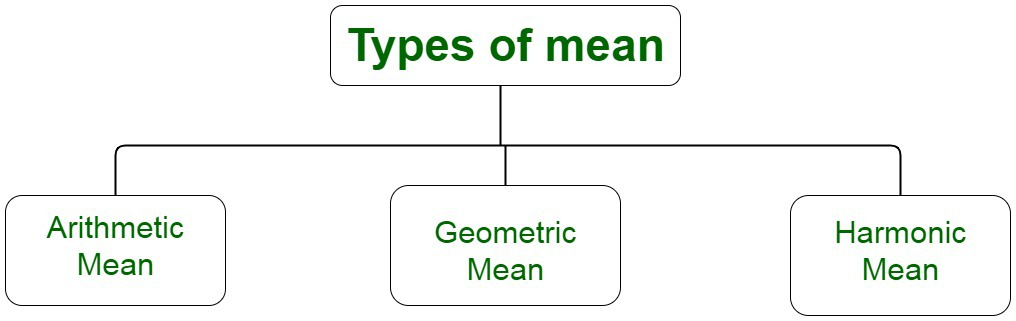# Find the mean of the first 10 natural numbers

• Last Updated : 06 Oct, 2021

Natural Numbers form the number system. They include all the positive integer numbers beginning with 1 up till infinity. They are also called counting numbers. Natural numbers don’t comprise integer zero or negative numbers. Decimal numbers and negative integer values are not accommodated in natural numbers. Natural numbers are a part of real numbers. Some of the examples of natural numbers are 23, 67, 89 etc.

### Mean

Mean is one of the statistical concepts which is used to calculate the average or a central value of a given set of integers. It gives information about the central tendency of the data. The central tendency of the data is a statistical measure that uses a single value to gather information about the entire set of data distribution. It provides a description of the entire data set.

Attention reader! All those who say programming isn't for kids, just haven't met the right mentors yet. Join the  Demo Class for First Step to Coding Coursespecifically designed for students of class 8 to 12.

The students will get to learn more about the world of programming in these free classes which will definitely help them in making a wise career choice in the future.

Mean is equivalent to the sum of all observations to the total number of observations. Mean is also called the arithmetic mean or average. Let us assume a data set to be given by, X = x1, x2,…, xn. The mean is denoted by x̄  which is the mean of given n values.

According to the definition, we have,

X̄ = Sum of Values / Number of Values

or

X̄ = (x1 + x2 + x3 + … + xn) / n### How do you find the mean of the first 10 natural numbers?

Natural numbers are counting numbers greater than 0.

We know, the first ten natural numbers are 1, 2, 3, 4, 5, 6, 7, 8, 9, 10.

The mean of n given numbers is the sum of the values of these n numbers divided by n. Computing the mean of the first ten natural numbers, we have,Solving the equation, we have,Thus, the mean of the numbers is 5.5.

### Similar Questions

Question 1: Where is the mean primarily used?

Solution :

Mean is a statistic measure, primarily used to the computation of statistic data in finance and marketing and conducting surveys.

Question 2: Compute the mean value of the first 10 even numbers.

We know, the first ten even numbers are 2, 4, 6, 8, 10, 12, 14, 16, 18, 20.

Mean of n given numbers is the sum of the values of these n numbers divided by n.

Then, the mean of these ten even numbers is 2, 4, 6, 8, 10, 12, 14, 16, 18, 20 is,Solving the equation, we have,Thus, the mean of the numbers is 11.

Question 3 : Let us assume that the heights of five given people are 142 cm, 150 cm, 149 cm, 156 cm, and 153 cm. Compute the mean height among them.

Computing the mean height, we have,Therefore, the computed mean height, x̄ =150 cm.

Question 4: The mean of five numbers, x , x+1, x+2 , x+3, x+4 is equivalent to 10 . Find x.Question 5: Guess the last number in a sequence of 3 numbers, 20, 34, x with a mean of 20.